• 数理逻辑

推理的形式结构

数理逻辑的主要任务是用数学的方法研究推理。所谓推理是指从前提出发推出结论的思维过程，而前提是已知的命题公式集合，结论是从前提出发应用推理规则推出的命题公式。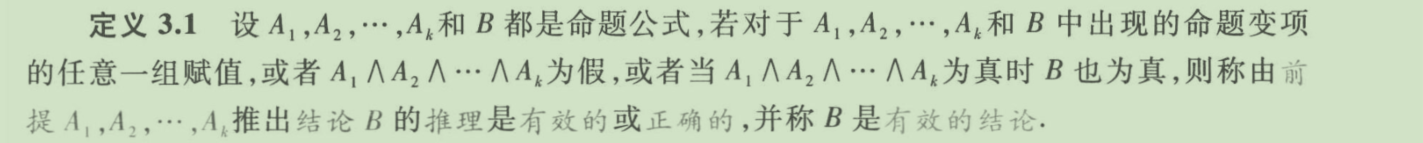推理的形式结构

前提： A 1 , A 2 , . . . , A k A_1,A_2,...,A_k
结论：B

判断是否为重言式的三种方法：
1、真值表法
2、等值演算法
3、主析取范式法

9条推理定律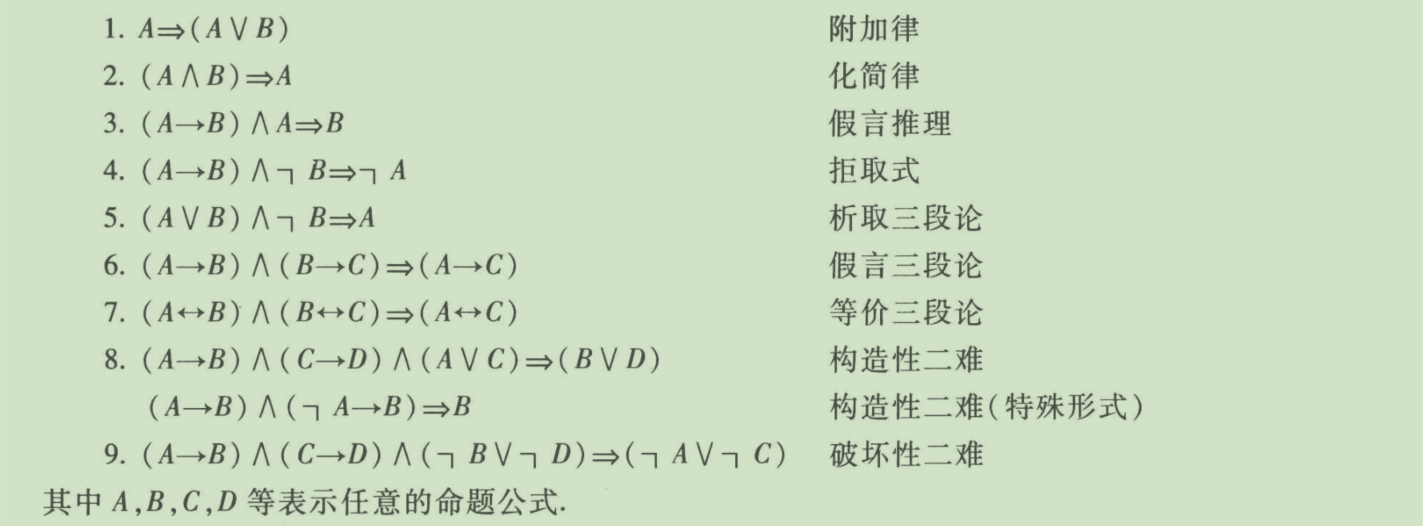自然推理系统P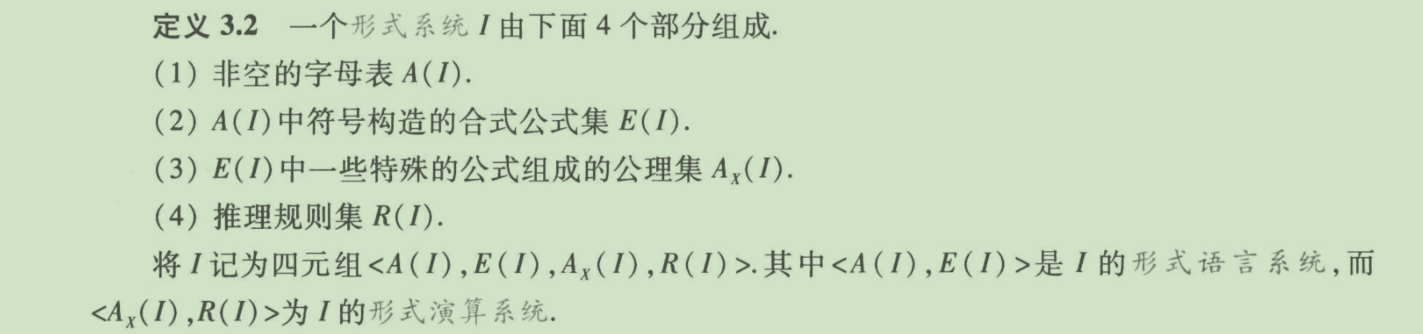自然推理系统P定义如下：
1、字母表：
（1）命题变项符号： p , q , r , . . . , p i , q i , r i , . . . p,q,r,...,p_i,q_i,r_i,...
（2）联结词符号： ¬ , ∧ , ∨ , → , ↔ \lnot ,\land,\lor,\to,\leftrightarrow
（3）括号与逗号：(，)，，
2、合式公式：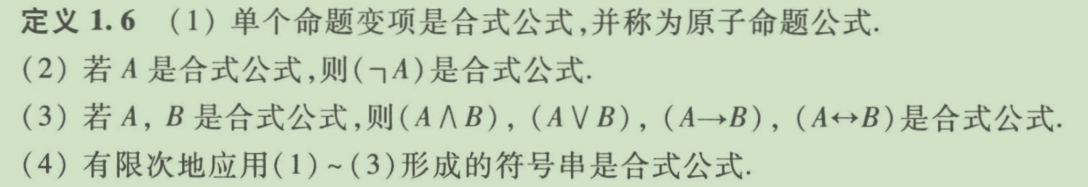3、推理规则：
（1） 前提引入规则
（2） 结论引入规则
（3） 置换规则
（4） 假言推理规则（或分离规则）
（5） 附加规则
（6） 化简规则
（7） 拒取式规则
（8） 假言三段论规则
（9） 析取三段论规则
（10）构造性二难推理规则
（11）破坏性二难推理规则
（12）合取引入规则

构造证明的方法
1、附加前提证明法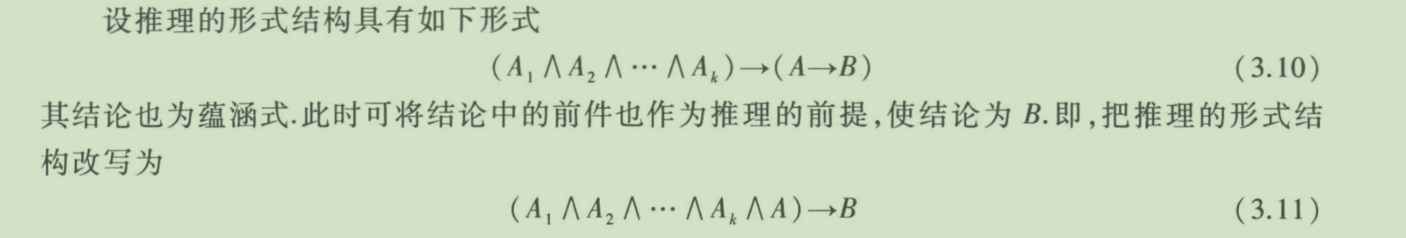2、归谬法展开全文• 命题逻辑推理理论 一些笔记： 判断推理是否正确，就是判断是否会出现1推出0的情况，如出现则推理错误，否则正确。 命题公式A1,A2,...,AkA_1,A_2,...,A_kA1​,A2​,...,Ak​推出B的推理正确当且仅当 A1∧A2∧...,∧...

命题逻辑的推理理论

一些笔记：

判断推理是否正确，就是判断是否会出现1推出0的情况，如出现则推理错误，否则正确。

命题公式 A 1 , A 2 , . . . , A k A_1,A_2,...,A_k 推出B的推理正确当且仅当

A 1 ∧ A 2 ∧ . . . , ∧ A k → B A_1 \wedge A_2 \wedge...,\wedge A_k \rightarrow B

为永真式。

判断推理是否正确的暴力方法，真值表法。

9条推理定律

附加律

A ⇒ ( A ∨ B ) A \Rightarrow(A \vee B)

化简律

( A ∧ B ) ⇒ A (A \wedge B) \Rightarrow A

假言推理

( A → B ) ∧ A ⇒ B (A \rightarrow B) \wedge A \Rightarrow B

拒取式

( A → B ) ∧ ¬ B ⇒ ¬ A (A \rightarrow B) \wedge \neg B \Rightarrow \neg A

析取三段论

( A ∨ B ) ∧ ¬ B ⇒ A (A \vee B) \wedge \neg B \Rightarrow A

假言三段论

( A → B ) ∧ ( B → C ) ⇒ ( A → C ) (A \rightarrow B) \wedge(B \rightarrow C) \Rightarrow(A \rightarrow C)

等价三段论

( A ↔ B ) ∧ ( B ↔ C ) ⇒ ( A ↔ C ) (A \leftrightarrow B) \wedge(B \leftrightarrow C) \Rightarrow(A \leftrightarrow C)

构造性二难

( A → B ) ∧ ( C → D ) ∧ ( A ∨ C ) ⇒ ( B ∨ D ) (A \rightarrow B) \wedge(C \rightarrow D) \wedge(A \vee C) \Rightarrow(B \vee D)

构造性二难（特殊形式）

( A → B ) ∧ ( ¬ A → B ) ∧ ( A ∨ ¬ A ) ⇒ B (A \rightarrow B) \wedge(\neg A \rightarrow B) \wedge(A \vee \neg A) \Rightarrow B

破坏性二难

( A → B ) ∧ ( C → D ) ∧ ( ¬ B ∨ ¬ D ) ⇒ ( ¬ A ∨ ¬ C ) (A \rightarrow B) \wedge(C \rightarrow D) \wedge(\neg B \vee \neg D) \Rightarrow(\neg A \vee \neg C)

推理规则

除上面9条推理定律所推出来的规则外，还有

合取引入规则：

A B ∴ A ∧ B \begin{array}{c} \boldsymbol{A} \\ \boldsymbol{B} \\ \hline \therefore A \wedge \boldsymbol{B} \end{array}

自然推理系统P

直接证明：就是通过前提加推理规则推出结论。

附加前提证明法：如结论中存在 A → B A \rightarrow B 形式，可以将A作为前提来使用。

归谬法：将结论的否定作为条件，推出矛盾式，则推理正确。

消解证明法：将公式和结论的否定化成等值的合取范式，通过消解得到空式。除准备工作外，全程只能用前提引入以及消解两条规则。

展开全文离散数学 命题逻辑 推理理论
• 命题逻辑推理

2021-11-03 13:25:49
命题逻辑推理 （1）实现命题逻辑的语法检查功能：合式公式的判定 （2）判断命题公式是否可满足； （3）判断命题公式的蕴含关系是否成立。 参考链接：C语言中缀表达式转后缀表达式 离散数学判断合式公式，并打印真值...

命题逻辑推理
（1）实现命题逻辑的语法检查功能：合式公式的判定
（2）判断命题公式是否可满足；
（3）判断命题公式的蕴含关系是否成立。
参考链接：C语言中缀表达式转后缀表达式
离散数学判断合式公式，并打印真值表、求主析取、主合取范式

#include<iostream>
#include<stdio.h>
#include <set>
#include <map>
#include <windows.h>
#include <stack>
using namespace std;
stack<char> s3;//栈s3存储后缀表达式
map<char, bool>ch;//映射表示原子命题真假
set<char>sh;//统计原子命题
//定义联结词优先次序
//case '!':return 5; break;
//case '&':return 4; break;
//case '|':return 3; break;
//case '-':return 2; break;
//case '=':return 1; break;
int Compare(char str1) {
if (str1 == '!') {
return 5;
}
else if (str1 == '&') {
return 4;
}
else if (str1 == '|') {
return 3;
}
else if (str1 == '-') {
return 2;
}
else if (str1 == '=') {
return 1;
}
else if (str1 == '(' || str1 == ')') {
return 0;
}
else {
return -1; //字母或者数字
}
}
bool Fouding(bool a)//否定运算
{
if (a)return false;
else return true;
}
bool And(bool a, bool b)//合取
{
if (!a || !b)return false;
else return true;
}
bool Or(bool a, bool b)//析取
{
if (a || b)return true;
else return false;
}
bool One(bool a, bool b)//条件
{
if (a && !b)return false;
else return true;
}
bool Two(bool a, bool b)//双条件
{
if (a == b)return true;
else return false;
}

void appoint(int num)
{
for (set<char>::iterator it = sh.begin(); it != sh.end(); it++)//遍历原子命题
{
if (num % 2)
ch[*it] = true;
else
ch[*it] = false;
num /= 2;
}
}

int rps(string& str, string ss, string sd, int runum)//用"sd"替换"str"中的"ss"
{

int i = str.length(), p;
while (p = str.find(ss) + 1)
str.replace(p - 1, ss.length(), sd);

return str.length() - i;
}
bool judge(string& str)//判断是否为合式公式
{
string strtmp = str;
for (int i = 0; i < str.length(); i++)
if (isalpha(str[i]))
str[i] = '1';

while (rps(str, "!1", "1", 2) || rps(str, "(1)", "1", 2) || rps(str, "1&1", "1", 3) || rps(str, "1|1", "1", 3) || rps(str, "1-1", "1", 3) || rps(str, "1=1", "1", 3));//使用规则2、3
if (str == "1")
{
cout << strtmp << "是合式公式" << endl;
return true;
}
else
{
cout << strtmp << "不是合式公式" << endl;
return false;
}
}
//∧∨→ <=>
void turn(string& s)
{
stack<char>s1, s2;
for (int i = 0; i < s.size(); i++)
{
char p = s[i];
if (isalpha(p))
s1.push(p);         //原子命题直接压入s1
else if (p == '(')      //左括号直接压入s2
s2.push(p);
else if (p == ')')
{
//遇右括号将s2中左括号前面的元素依次弹出压入s1
while (!s2.empty() && s2.top() != '(')
{
s1.push(s2.top());
s2.pop();
}
s2.pop();
}
else        //遇到逻辑符号
{
if (s2.empty())
s2.push(p);
else if (s2.top() == '(')
s2.push(p);
else if (Compare(p) > Compare(s2.top()))
s2.push(p);
else
{
while (!s2.empty() && s2.top() != '(' && Compare(p) <= Compare(s2.top()))
{
s1.push(s2.top());
s2.pop();
}
s2.push(p);
}
}
}
while (!s2.empty())//将s2中剩余元素压入s1
{
s1.push(s2.top());
s2.pop();
}

while (!s1.empty())//将后缀表达式存储在栈s3中
{
s3.push(s1.top());
s1.pop();
}

}
int  value()//后缀表达式求值
{
ch['T'] = true;
ch['F'] = false;
stack<char>c, s;
s = s3;
while (!s.empty())
{
char p = s.top();//取栈顶元素
s.pop();
if (isalpha(p))
c.push(p);//原子命题直接压入c
else if (p == '!')//联结词!处理
{
char t = c.top();
c.pop();
Fouding(ch[t]) ? c.push('T') : c.push('F');
}
else
{
char ph;
char t1 = c.top();
c.pop();
char t2 = c.top();
c.pop();
switch (p)
{
case '&':And(ch[t2], ch[t1]) ? ph = 'T' : ph = 'F'; break;
case '|':Or(ch[t2], ch[t1]) ? ph = 'T' : ph = 'F'; break;
case '-':One(ch[t2], ch[t1]) ? ph = 'T' : ph = 'F'; break;
case '=':Two(ch[t2], ch[t1]) ? ph = 'T' : ph = 'F'; break;
}
c.push(ph);
}
}
if (c.top() == 'T')  return true;
else return false;
}
int judge2(int cou)
{
for (int i = 0; i < cou; i++)//打印真值表
{
appoint(i);//指派
for (set<char>::iterator it = sh.begin(); it != sh.end(); it++)
{
if (value() == 1)
{
return 1;
}
else continue;
}
}
return 0;
}
int judge3(int cou)
{
for (int i = 0; i < cou; i++)//打印真值表
{
appoint(i);//指派
for (set<char>::iterator it = sh.begin(); it != sh.end(); it++)
{
if (value() == 0)
{
return 0;
}
else
continue;
}
}
return 1;
}
printf("*************************************************\n");
printf("*\t欢迎使用命题逻辑推理系统V1.0\t\t*\n");
printf("*\t\t请选择功能\t\t\t*\n");
printf("*************************************************\n");
printf("*\t0.退出命题逻辑推理系统\t\t\t*\n");
printf("*\t1.判定命题公式是否为合式公式\t\t*\n");
printf("*\t2.判断命题公式是否可满足\t\t*\n");
printf("*\t3.判断命题公式的蕴含关系是否成立\t*\n");
printf("*************************************************\n");
}

int main()
{

string str, input;
cout << "请输入合式公式(!否定 , 合取 & ， 析取 | , 条件 - ,双条件 = )：" << endl;
while (1)
{
int choice;
cin >> choice;
switch (choice)
{
case 0:
{
cout << "感谢你使用命题逻辑推理系统,,请关掉程序!!!" << endl;
system("pause");
}break;
case 1:
{
cin >> str;
judge(str);
}break;
case 2:
{
cin >> str;
for (int i = 0; i < str.size(); i++)//将原子命题建立到set容器中
if (isalpha(str[i]))sh.insert(str[i]);
turn(str);//将前缀表达式转化为后缀表达式
int cou = pow(2, sh.size());
if (judge2(cou) == 1)
{
cout << "命题公式满足" << endl;
}
else
cout << "命题公式不满足" << endl;

}break;
case 3:
{
cin >> str;
for (int i = 0; i < str.size(); i++)//将原子命题建立到set容器中
if (isalpha(str[i]))sh.insert(str[i]);
turn(str);//将前缀表达式转化为后缀表达式
int cou = pow(2, sh.size());
if (judge3(cou) == 1)
{
cout << "命题公式的蕴含关系成立" << endl;
}
else
cout << "命题公式的蕴含关系不成立" << endl;
}break;
default:
{
printf("选择错误，请重新输入0.0\n");
}break;
}
}
system("pause");
return 0;

}

展开全文数据结构 字符串
• 一、 命题逻辑推理正确性判定 、 二、 形式结构是永真式 ( 等值演算 ) 、 三、 从前提推演结论 ( 逻辑推理 ) 、

一、 命题逻辑推理正确性判定

命题推理 , 根据 前提 , 推理出 结论 ;

如 :
前提 : p → ( q → r ) p \to (q \to r) , p p , q q ;
结论 : r r

如何判定根据上述前提 , 推理出的结论是正确的呢 ?

推理定律 : A , B A,B 是两个命题 , 如果 A → B A \to B 是永真式 , 那么 A ⇒ B A \Rightarrow B ;

推理的形式结构

前提 : A 1 , A 2 , ⋯   , A k A_1 , A_2 , \cdots , A_k

结论 : B B

推理的形式结构为 : ( A 1 ∧ A 2 ∧ ⋯ ∧ A k ) → B (A_1 \land A_2 \land \cdots \land A_k) \to B

命题逻辑 推理的正确性 判定 , 有两种方法 ;

方法一 : 写出推理的 形式结构 , 查看该推理的形式结构是不是 永真式 ; 如果是永真式 , 那么该推理是正确的 ;

方法二 :前提 推演 结论 , 根据 等值演算规则 , 推理规则 , 进行推演 ;

二、 形式结构是永真式 ( 等值演算 )

前提 : p → ( q → r ) p \to (q \to r) , p p , q q ;
结论 : r r

推理的形式结构是 : ( p → ( q → r ) ) ∧ p ∧ q → r (p \to (q \to r)) \land p \land q \to r

使用 等值演算 的方法 , 验证上述形式结构是否是 永真式 ;

联结词的 优先级为 : ¬ \lnot 大于 ∧ , ∨ \land , \lor 大于 → , ↔ \to, \leftrightarrow ; 先从优先级较高的开始进行 ;

( p → ( q → r ) ) ∧ p ∧ q → r (p \to (q \to r)) \land p \land q \to r

蕴涵等值式 : 使用 蕴涵等值式 规则 , 将上述 ( p → ( q → r ) ) (p \to (q \to r)) 进行等值演算 :

⇔ ( ¬ p ∨ ( ¬ q ∨ r ) ) ∧ p ∧ q → r \Leftrightarrow (\lnot p \lor (\lnot q \lor r)) \land p \land q \to r

分配率 : 根据 分配率 , 计算 ( ¬ p ∨ ( ¬ q ∨ r ) ) ∧ p (\lnot p \lor (\lnot q \lor r)) \land p 部分 :

⇔ ( ( ¬ p ∧ p ) ∨ ( ( ¬ q ∨ r ) ∧ p ) ) ∧ q → r \Leftrightarrow (( \lnot p \land p ) \lor ( (\lnot q \lor r) \land p ) ) \land q \to r

矛盾律 : 其中 根据 矛盾律 可知 , ¬ p ∧ p ⇔ 0 \lnot p \land p \Leftrightarrow 0 :

⇔ ( 0 ∨ ( ( ¬ q ∨ r ) ∧ p ) ) ∧ q → r \Leftrightarrow ( 0 \lor ( (\lnot q \lor r) \land p ) ) \land q \to r

同一律 : 根据 同一律 , 0 ∨ ( ( ¬ q ∨ r ) ∧ p ) 0 \lor ( (\lnot q \lor r) \land p ) ( ¬ q ∨ r ) ∧ p (\lnot q \lor r) \land p 是等价的 :

⇔ ( ( ¬ q ∨ r ) ∧ p ) ∧ q → r \Leftrightarrow ( (\lnot q \lor r) \land p ) \land q \to r

结合律 : 根据 结合律 , 重新结合 ( ( ¬ q ∨ r ) ∧ p ) ∧ q ( (\lnot q \lor r) \land p ) \land q ( ( ¬ q ∨ r ) ∧ q ) ∧ p ( (\lnot q \lor r) \land q ) \land p :

⇔ ( ( ¬ q ∨ r ) ∧ q ) ∧ p → r \Leftrightarrow ( (\lnot q \lor r) \land q ) \land p \to r

分配率 : 根据 分配率 , 计算 ( ¬ q ∨ r ) ∧ q (\lnot q \lor r) \land q , 结果是 ( ¬ q ∧ q ) ∨ ( r ∧ q ) (\lnot q \land q) \lor (r \land q)

⇔ ( ( ¬ q ∧ q ) ∨ ( r ∧ q ) ) ∧ p → r \Leftrightarrow ( (\lnot q \land q) \lor (r \land q) ) \land p \to r

矛盾律 : 根据 矛盾律 计算 ¬ q ∧ q \lnot q \land q , 其结果是 0 0 :

⇔ ( 0 ∨ ( r ∧ q ) ) ∧ p → r \Leftrightarrow ( 0 \lor (r \land q) ) \land p \to r

同一律 : 根据同一律 , 0 ∨ ( r ∧ q ) 0 \lor (r \land q) 等价于 ( r ∧ q ) (r \land q) :

⇔ ( r ∧ q ) ∧ p → r \Leftrightarrow (r \land q) \land p \to r

联结词优先级 : ( r ∧ q ) ∧ p (r \land q) \land p 中 , 联结词优先级相同 , 括号可以删除 , 将三个命题放在一个括号中 ;

⇔ ( r ∧ q ∧ p ) → r \Leftrightarrow (r \land q \land p ) \to r

蕴涵等值式 : 根据 蕴涵等值式 , 消去 蕴涵联结词 → \to :

⇔ ¬ ( r ∧ q ∧ p ) ∨ r \Leftrightarrow \lnot (r \land q \land p) \lor r

德摩根律 : 根据 德摩根律 , 将否定符号分配到括号中 ;

⇔ ( ¬ r ∨ ¬ q ∨ ¬ p ) ∨ r \Leftrightarrow (\lnot r \lor \lnot q \lor \lnot p ) \lor r

联结词优先级 : ( ¬ r ∨ ¬ q ∨ ¬ p ) ∨ r (\lnot r \lor \lnot q \lor \lnot p ) \lor r 中 , 联结词优先级相同 , 括号可以删除 , 将三个命题放在一个括号中 ;

⇔ ¬ r ∨ ¬ q ∨ ¬ p ∨ r \Leftrightarrow \lnot r \lor \lnot q \lor \lnot p \lor r

排中律 : 根据排中律 , ¬ r ∨ r \lnot r \lor r 1 1 等价 ;

⇔ 1 ∨ ¬ q ∨ ¬ p \Leftrightarrow 1 \lor \lnot q \lor \lnot p

零律 : 根据零律 , 1 1 析取任何值 , 都等价于 1 1 :

⇔ 1 \Leftrightarrow 1

三、 从前提推演结论 ( 逻辑推理 )

前提 : p → ( q → r ) p \to (q \to r) , p p , q q ;
结论 : r r

将前提条件使用合取联结词连接起来 , ( p → ( q → r ) ) ∧ p ∧ q (p \to (q \to r)) \land p \land q , 进行等值演算 , 计算出 r r ;

( p → ( q → r ) ) ∧ p ∧ q (p \to (q \to r)) \land p \land q

等值演算 结合律 :

⇔ ( ( p → ( q → r ) ) ∧ p ) ∧ q \Leftrightarrow ((p \to (q \to r)) \land p) \land q

逻辑推理 假言推理 : ( A → B ) ∧ A ⇒ B ( A \to B ) \land A \Rightarrow B , 因此从 ( p → ( q → r ) ) ∧ p (p \to (q \to r)) \land p 可以推理出 q → r q \to r ;

⇒ ( q → r ) ∨ q \Rightarrow (q \to r) \lor q

逻辑推理 假言推理 : ( A → B ) ∧ A ⇒ B ( A \to B ) \land A \Rightarrow B , 因此从 ( q → r ) ∨ q (q \to r) \lor q 可以推理出 r r ;

⇒ r \Rightarrow r

逻辑推理 比 等值演算 快 , 等值演算比较直观 , 逻辑推理需要选择合适的推理定律 ;

展开全文等值演算 逻辑推理 命题逻辑 离散数学
• 命题逻辑的一些推理规则

千次阅读 2009-12-26 16:34:00
2、逆否命题法则：(~表示非) p-&gt;q ∴~q-&gt;~p 3、拒取法则： p-&gt;q ~q ∴~p 4、链规则（三段论法则） p-&gt;q q-&gt;r ∴p-&gt;r 5、析取推理法则 p∨q ~p ∴q p∨q ...
• 命题逻辑推理理论 置换规则 A=B⇒(A⇒B) A=B\Rightarrow (A\Rightarrow B) A=B⇒(A⇒B) 假言推理规则 p,p→q⇒q p,p\rightarrow q\Rightarrow q p,p→q⇒q 附加规则 p⇒p∨q p\Rightarrow p\vee q p⇒p∨q ...逻辑推理
• 若前提为k个命题公式：A1,A2,A3,...,AkA_1,A_2,A_3,...,A_kA1​,A2​,A3​,...,Ak​，结论为命题公式BBB，对命题公式赋值时，前提和结论的取值情况有以下4种： A1∧A2∧...∧AkA_1\land A_2\land ...\land A_kA1​...离散数学
• 在基于格值逻辑的不确定性推理的研究中，推理规则的...基于分层格值命题逻辑系统，提出了几类既包含有语义又含有语法的推理规则，且这些推理规则具备协调水平的特性；同时也证明了这几类推理规则在一定程度上有闭性。
• 第三章命题逻辑推理理论 1.推理的形式结构 （1）定义3.1：设A1，A2，A3...Ak和B都是命题公式，若对于A1，A2，A3...Ak和B中出现的命题变项的任意一组赋值，或者A1，A2，A3...Ak为假，或者当A1，A2，A3...Ak为真是，...数学 离散数学
• Natural Logic Interpreter根据推理规则命题逻辑自动解释和验证嵌套的自然逻辑论证（以自然语言表达的逻辑论证）。 该程序使用递归函数实现的“分而治之”算法来遍历嵌套的逻辑参数，并能够相应地定义和验证它们。...
• 引言 数理逻辑：是用数学方法来研究推理的形式结构和推理规律的数学学科。 推理：从前提推出结论的思维过程。...结论：指从前提出发应用推理规则推出的命题。 推理定律——重言蕴含式      ...
• 给出了相干命题逻辑自然推理系统NR的自动证明算法。首先将待证命题公式A的子公式组成一个初始集合P，对其中的元素采用系统NR的推理规则得到新的命题公式加入P，当得到秩为0的A时命题得证；然后对A的证明树进行整理即...
• 7.1 重要推理规则 在【离散数学】数理逻辑 第一章 命题逻辑(3) 逻辑等价与蕴含中列出的等价公式和蕴含公式，都可以作为推理规则使用。此外在推理中，还有两条常用的重要推理规则： P规则：在推理过程中，前提可以在...离散数学
• 定义： 称蕴含式（A1A_1A1​ ∧\wedge∧ A2A_2A2​ ∧\wedge∧ ⋯\cdots⋯ ∧\wedge∧ AnA_nAn​）→\rightarrow→ B 为推理的形式结构\red{推理的形式结构 }推理的形式结构，A1A_1A1​ , A2A_2A2​, ⋯\cdots⋯ ,...推理理论
• 文章目录一 推理的形式结构1 推理的形式结构2 推理定律-重言蕴涵式二 自然推理系统P推理规则直接证明法附加前提法归谬发（反证法） 一 推理的形式结构 1 推理的形式结构 2 推理定律-重言蕴涵式 二 自然推理系统P ...1024程序员节 命题逻辑
• Chapter Three - 命题逻辑推理理论 1 - 要点 推理 证明 推理的形式结构的符号化形式：A₁∧A₂∧…∧Ak→B（*） 如果（*）是重言式，则称推理是有效的，或称推理是正确的数理逻辑 命题逻辑
• (3) 用命题逻辑形式描述实现上述输入-输出过程所应用的判定规则，并写出获得输出结果的推理演算过程 第6列实现上述输入-输出过程所应用的判定规则 C1 ∧ \wedge ∧ T11 ⇒ \Rightarrow ⇒ T13 C2 ...命题逻辑推理
• 第三章命题逻辑的推理理论 3.1推理的形式结构 数理逻辑的主要任务是用数学的方法研究推理.所谓推理是指从前提出发推出结论的思维过程,而前提是已知的命题公式集合,结论是从前提出发应用推理规则推出的命题公式...
• 命题逻辑和谓词逻辑的异同

千次阅读 2020-04-25 10:49:08
转载自：... 关键词：命题逻辑、谓词逻辑、一阶谓词逻辑、 “所有个体”、“存在个体”中，量词加在论域的个体上，称为一阶量词。  在一阶逻辑中使用的量词...人工智能 命题逻辑 谓词逻辑
• 文章目录自然推理系统的定义13个推理规则如何在自然推理系统中构造有效论证的方法直接证明法附加前提证明法（cp规则）反证法 / 归谬法（Proofs by Contradiciton）对位证明（Proofs by contraposition）举例直接证明...
• 文章目录逻辑推理解推理问题的基本方法：判断推理是否正确的方法：判断一个推理形式是否正确，从定义上讲就是判断一个蕴含式是否是重言式数学证明与形式推理的区别：推论定律——重言蕴涵式推理规则构造证明法一、...线性代数 抽象代数
• 目录 一、写在前面 二、inference之形式推演deduction 三、第一个形式推演系统（11条规则） 四、什么叫形式推演（形式可...我们上一篇写了命题逻辑，和命题逻辑中语义逻辑推理entailment部分内容，我们知道学习...
• 定义3.1 设A1, A2, …, Ak, B为命题公式. 若对于每组赋值，A1A2…Ak 为假，或当A1A2…Ak为真时，B也为真，则称由前提A1, A2, …, Ak推出结论B的...定理3.1 由命题公式A1, A2, …, Ak 推B的推理
• 前面说完了自然演算规则，现在来说导出规则。   导出规则有四个，分别是：MT导出规则，双重否定引入规则，PBC导出规则，LEM导出规则。 记的的同学可能会问了：咦，前两个不是在自然演算规则里出现了吗？ 是的，...
• 数理逻辑、命题逻辑、谓词逻辑之概念详细梳理 一、前言 二、概念梳理 1、数理逻辑 （1）数理逻辑包括哪些内容呢？ （2）数理逻辑体系 2、命题逻辑 3、谓词逻辑 三、命题逻辑和谓词逻辑之间有什么关系？ 1、解释一： ...逻辑推理 概念数据模型
• 命题逻辑 语法规则 P::=T∣⊥∣x∣P∧P∣P∨P∣P→P∣¬P P::= T|⊥| x | P ∧P | P ∨ P | P → P | ¬ P P::=T∣⊥∣x∣P∧P∣P∨P∣P→P∣¬P 用符号P代表任意逻辑命题，它由几种不同语法形式组成:符号T和⊥分别...逻辑推理
• 命题逻辑详解

2021-03-12 19:26:21
命题逻辑详解 文章目录命题逻辑详解一.命题逻辑的基本概念1....命题逻辑推理理论1.推理的有效性2.命题逻辑的自然推理系统3.构造验证推理有效性的论证六.命题逻辑的应用1.自然语言命题的符号化2.普通逻辑问题的符号化
• 给出了直觉模糊逻辑命题的运算规则,重点研究了基于直觉模糊逻辑的近似推理方法.该方法包括直觉模糊取式推理,直觉模糊拒式推理及直觉模糊假言推理,并推导了相关的推理合成运算公式.以具体算例验证和表明了所提出的......